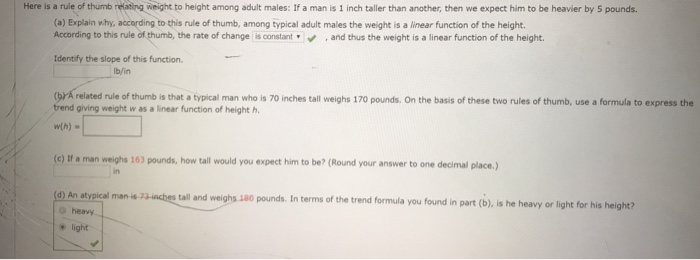# Here is a rule of thumb ing weight to height among adult males: If a man is 1 inch taller than another, then we expect him to be heavier by 5 pounds (a) Explain why, accarding to this rule of thumb, among typical adult males the weight is a linear function of the height. According to this rule of thumb, the rate of change is constantand thus the weight is a linear function of the height. Identify the slope of this function. lb/in (yr A related rule of thumb is that a typical man who is 70 inches tall weighs 170 pounds. On the basis of these two rules of thumb, use a formula to express the trend giving weight w as a linear function of height h. w(h). (c) If a man weighs 163 pounds, how tall would you expect him to be? (Round your answer to one decimal place,) in (d) An atvpical man is all and weighs 1a0 pounds. In terms of the trend formula you found in part (b), is he heavy or light for his height? heavy light

Question

Here is a rule of thumb relating weight to height among adult males : if a man is 1 inch taller than another, then we expect him to be heavier by 5 pounds.

-identify the slope of this function

(B) a related rule of thumb is that a typical man who is 70 inches tall weighs 170 pounds. On the basis of these two rules of thumb, use a formula to express the trend giving weight w as a linear function of height h. W(h)=_______________

(C) if a man weighs 163 pounds, how tall would you expect him to be? _________ incheshelp_outlineImage TranscriptioncloseHere is a rule of thumb ing weight to height among adult males: If a man is 1 inch taller than another, then we expect him to be heavier by 5 pounds (a) Explain why, accarding to this rule of thumb, among typical adult males the weight is a linear function of the height. According to this rule of thumb, the rate of change is constantand thus the weight is a linear function of the height. Identify the slope of this function. lb/in (yr A related rule of thumb is that a typical man who is 70 inches tall weighs 170 pounds. On the basis of these two rules of thumb, use a formula to express the trend giving weight w as a linear function of height h. w(h). (c) If a man weighs 163 pounds, how tall would you expect him to be? (Round your answer to one decimal place,) in (d) An atvpical man is all and weighs 1a0 pounds. In terms of the trend formula you found in part (b), is he heavy or light for his height? heavy light fullscreen

### Want to see the step-by-step answer?